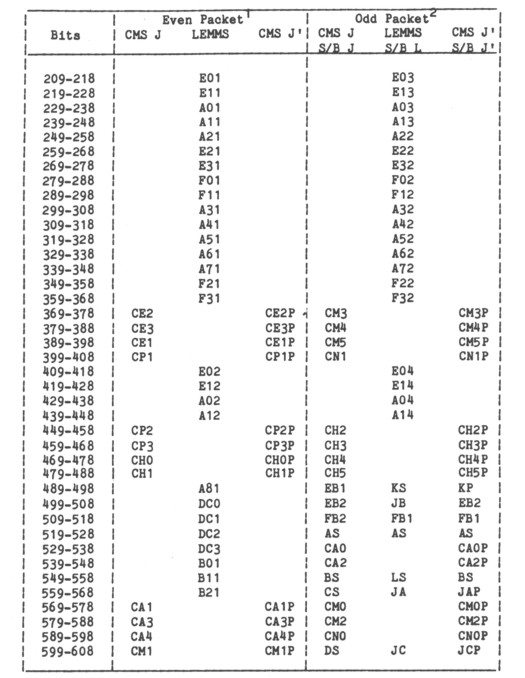GALILEO

## Galileo EPD Handbook

### Appendices

Appendix A. EDR File Structure (continued)

3. EPD Subsystem Telemetry (continued)

Source: GLL-3-280, Rev. B, Appendix A, March 15, 1985

Rate Channel Data

The Rate Channel Data section contains 40 rate channels, 10 bits each, of CMS and LEMMS sensor data. The particular rate channel involved depends on the MOD 2 counter described in Table 2. Table 7 shows the contents of the Rate Channel section for odd and even packets.

EPD rate channel accumulators are 10 bits log compressed from 24 bits. The log compression algorithm can be stated as follows:

Given a 24 bit binary integer with MSB on the left and LSB on the right, find the most significant "1." The number of bits to the right of the most significant "1," minus 6, is the exponent. If this exponent value is negative, or if the original number itself is zero, set the exponent to 15, and use the 6 least significant bits of the original 24 bit number as the mantissa. If this is not the case, use the 6 bits immediately to the right of the most significant "1" as the mantissa.

The log decompression algorithm can be stated as follows:

Given the 10 bit log compressed rate channel data, the first 4 bits are the exponent and the last 6 bits are the mantissa. If the exponent = 15, then the value = mantissa; if not, then the value = (mantissa + 64) · 2exp.

Table 7. EPD Rate Channel DataNotes:
1. If the LEMMS column is blank, use the CMS J column if the J/J' indicator is zero, otherwise use the CMS J' column.
2. If the LEMMS S/B L column is blank, treat as in footnote 1. If all 3 columns contain names, this is a Singles/Background channel. Use the LEMMS S/B L column if the Singles/Background Flag is zero, otherwise treat the same as in footnote 1.